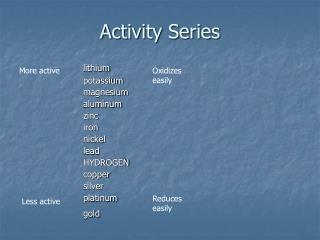DownloadDownload PresentationActivity Series

# Activity Series

Télécharger la présentation## Activity Series

- - - - - - - - - - - - - - - - - - - - - - - - - - - E N D - - - - - - - - - - - - - - - - - - - - - - - - - - -
##### Presentation Transcript

1. Activity Series More active Oxidizes easily lithium potassium magnesium aluminum zinc iron nickel lead HYDROGEN copper silver platinum gold Reduces easily Less active

2. Activity series • More active metals will displace less active metal ions. • Hydrogen is the reference point. • Electrons will flow spontaneously from the stronger reducing agent to the stronger oxidizing agent.

3. lithium potassium magnesium aluminum zinc iron nickel lead HYDROGEN copper silver platinum gold Will the following reactions occur ? Ag+ + Cu Cu2+ + Ag Mg2+ + Zn Zn2+ + Mg Zn2+ + Cu Mg2+ + Li Yes No No Yes No Yes The metal must be above (more active than) the ion for it to be a spontaneous reaction.

4. Electrochemistry Chemical energy becoming electrical energy

5. Current- the flow of electricity (amps) • Potential difference- the force pushing the current (volts) • Electrolyte- an ionic solution that can carry electric current. • Spontaneous redox reactions produce this electric energy.

6. Voltaic Cell Anode • attracts anions • where oxidation occurs Cathode • attracts cations • where reduction occurs Salt bridge • connects the two half cells • contains a strong electrolyte

7. Zn Zn+ Cu2+ Cu in shorthand Two half cells connected by a salt bridge

8. Reduction half reactions F2 is the strongest oxidizing agent Li is the strongest reducing agent

9. Reduction Potentials (E) • Eo is the standard electrode potential • all ions are 1M and gases are 1 atm • The net Eo is the sum of the Eo of the half reactions • The stronger oxidizing agent reduces. • Reverse the sign of the substance oxidized. • If E is positive, the reaction is spontaneous. • If E is negative, the reverse reaction is spontaneous.

10. What is the voltage produced from the reaction of Zn metal with Cu2+ ions? Zn(s) + Cu2+(aq) g Zn2+(aq) + Cu(s) Zn2+ + 2e-g Zn -0.7628 Cu2+ + 2e-g Cu 0.3402 -(-0.7628) + 0.3402 = 1.103 volts Will happen spontaneously Zn2+ + Ni(s) g Zn(s) + Ni2+ -(-0.23) + (-0.7628) = -.53 Will not occur spontaneously Cu2+ will reduce!

11. Faraday’s Law • Coulombs = amperes x seconds • 1 C = 1amp·1sec • 96,485 coulombs = 1 mole e-

12. What mass of copper will be deposited by a current of 7.89 amps flowing for 1200 seconds? • Cu2+ + 2e-g Cu at the cathode 7.89A x 1200s x 1C x 1 mol e-= .0981 mol e- A·s 96,485C .0981 mol e- x 1 mol Cux63.5g Cu = 3.1g Cu 2 mol e- 1 mole Cu

13. What mass of Cr3+ is produced by a current of 0.713A flowing for 12,800 seconds? 14H3O++ 6Fe2++ Cr2O72-g 6Fe3++ 2Cr3-+21H2O .713A x 12,800s x 1C x 1 mol e-= .0946 mol e- A·s 96,485C .0946 mol e-x2mol Cr3+x 52.0g Cr3+ = 1.64gCr3+ 6mol e- 1mol Cr3+

14. Nernst Equation • Eo Voltage under standard conditions (1M solutions at 25°C and 101.3kPa) At non-standard conditions, use Nernst equation E = E° - 0.05916 log [products] n [reactants] n = no. of electrons transferred Coefficients in front of reactants or products are used as powers of their concentrations.

15. Determine the voltage of a Zn Zn2+ (.75M) Ni2+(2.0M) Ni cell at standard temperature and pressure. Eo = +0.7626V + -.257V = .506V E = .506V - .0592 log .75M 2 2.0M E = .506V – 0.0296 (log 0.375) E = .506V – (-0.0126) E = .519V